# 1 Objectives

Here we will do classical linear regression in both frequentist and Bayesian setting. We will also show the difference between credible intervals and prediction intervals.

In Part 1 the data and the model are introduced and the traditional frequentist aproaches are described. Participants then try to implement the model in BUGS. In Part 2 the solution is exposed, and the difference between credible and prediction intervals is explained.

# 2 The data

We will use data from Michael Crawley’s R Book, Chapter 10 (Linear Regression). The data show the growth of catepillars fed on experimental diets differing in their tannin contnent.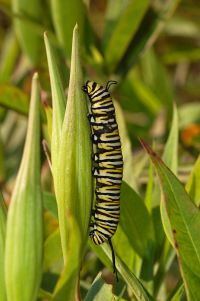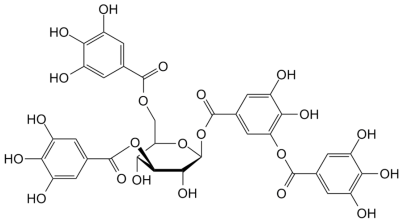To load the data to R directly from the web:

  catepil <- read.csv("https://rawgit.com/petrkeil/ML_and_Bayes_2017_iDiv/master/Linear%20Regression/catepilar_data.csv")
catepil
##   growth tannin
## 1     12      0
## 2     10      1
## 3      8      2
## 4     11      3
## 5      6      4
## 6      7      5
## 7      2      6
## 8      3      7
## 9      3      8

The data look like this:

  plot(growth~tannin, data=catepil, pch=19)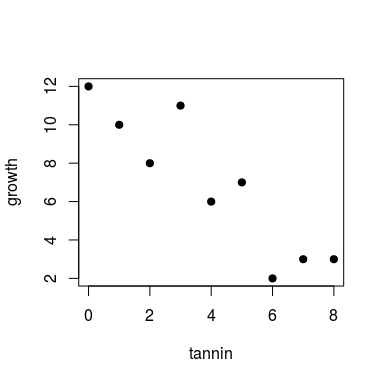# 3 The model

The classical notation: $growth_i = a + b \times tannin_i + \epsilon_i$ $\epsilon_i \sim N(0, \sigma)$

The notation that we will use:

$\mu_i = a + b \times tannin_i$ $growth_i \sim N(\mu_i, \sigma)$

These notations are mathematically equivalent, but the second notation shows more directly how we think about the stochastic part of the model. It is also more broadly used in the fields of hierarchical modelling and Bayesian analysis.

This is how Kurschke plots it (Kruschke (2014) Doing Bayesian Data Analysis, 2nd edition, Academic Press / Elsevier):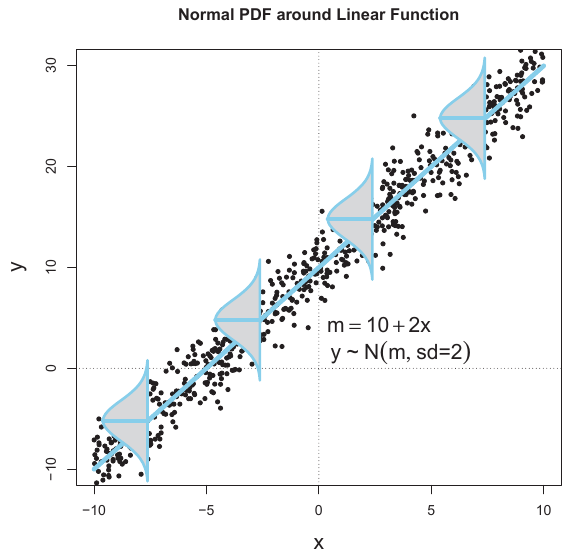# 4 Linear regression manually

This will work for those with R-studio only!

source("https://rawgit.com/petrkeil/ML_and_Bayes_2017_iDiv/master/Linear Regression/linear_regression_part0_functions.r")
## Loading required package: manipulate
manipulate(
regr.plot(x=catepil$tannin, y=catepil$growth, a, b, sigma),
a = slider(min=0, max=15, step=0.01, initial=5),
b = slider(min=-5, max=5, step=0.01, initial=-2),
sigma = slider(min=0, max=3, step=0.01, initial=0.1)
)

# 5 Linear regression fitted with MLE and optim

First we will define function returning value that we will optimize (i.e. minimize):

neg.LL.function.for.optim <- function(par, dat)
{
tannin <- dat$tannin growth <- dat$growth
a <- par
b <- par
sigma <- par

# likelihood
mu <- a + b*tannin
L <- dnorm(growth, mean=mu, sd=sigma)

# negative log-likelihood
neg.LL <- - sum(log(L))
return(neg.LL)
}

And here we use optim to do the actual optimization. Note: For 1-dimensional problems you can use optimize, or uniroot.

optim(par=c(a=0, b=0, sigma=1),
fn=neg.LL.function.for.optim,
dat=catepil)
## $par ## a b sigma ## 11.754421 -1.216341 1.492704 ## ##$value
##  16.37996
##
## $counts ## function gradient ## 206 NA ## ##$convergence
##  0
##
## \$message
## NULL

# 6 Linear regression using glm

  model.lm <- glm(growth~tannin, data=catepil)
  plot(growth~tannin, data=catepil, pch=19)
abline(model.lm)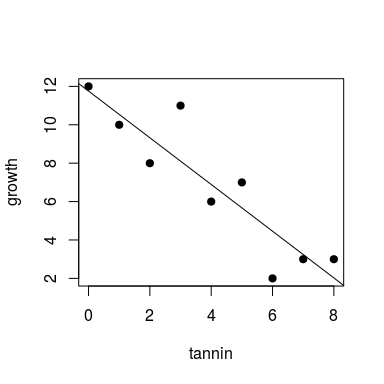summary(model.lm)
##
## Call:
## glm(formula = growth ~ tannin, data = catepil)
##
## Deviance Residuals:
##     Min       1Q   Median       3Q      Max
## -2.4556  -0.8889  -0.2389   0.9778   2.8944
##
## Coefficients:
##             Estimate Std. Error t value Pr(>|t|)
## (Intercept)  11.7556     1.0408  11.295 9.54e-06 ***
## tannin       -1.2167     0.2186  -5.565 0.000846 ***
## ---
## Signif. codes:  0 '***' 0.001 '**' 0.01 '*' 0.05 '.' 0.1 ' ' 1
##
## (Dispersion parameter for gaussian family taken to be 2.86746)
##
##     Null deviance: 108.889  on 8  degrees of freedom
## Residual deviance:  20.072  on 7  degrees of freedom
## AIC: 38.76
##
## Number of Fisher Scoring iterations: 2

And how about the $$\sigma$$?

# 7 Tasks for you

Using the ideas from previous lessons:

• Try to prepare the catepil data for this model in the list format.

• Try to write the regression model in the JAGS language and dump it into a file using cat.

• Try to fit the model in JAGS and estimate posterior distributions of $$a$$ and $$b$$.